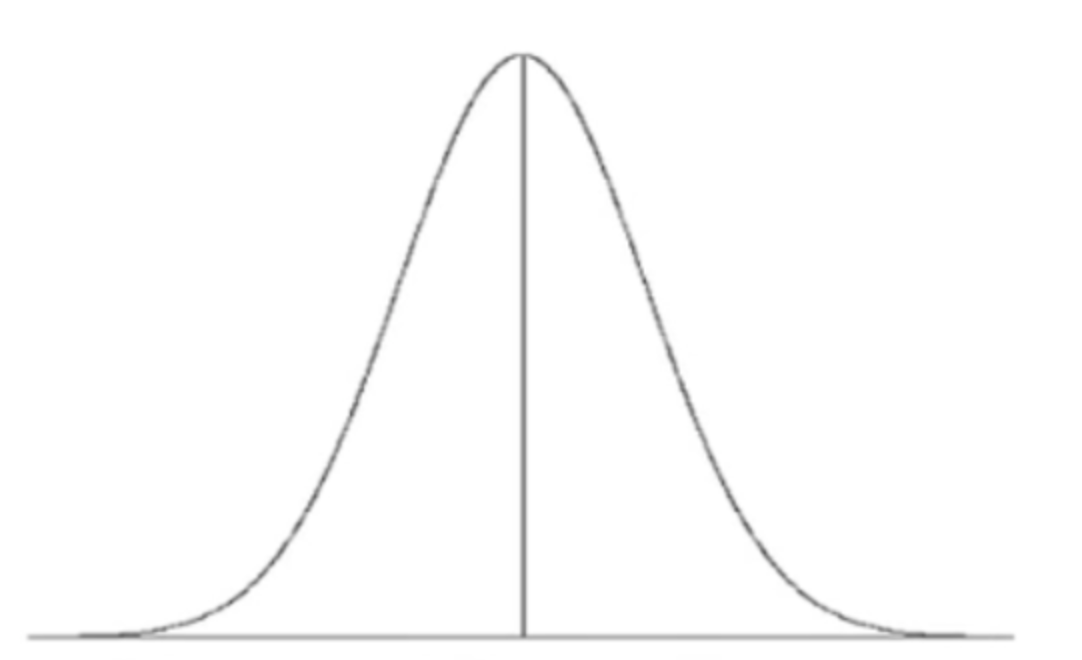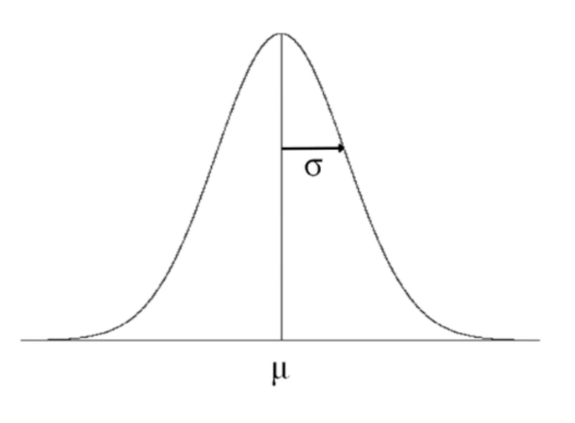+
Normal Distribution
Central Tendency, Variation, and Distributions
0
of 90 possible points
Common Core: S.ID.4

# Normal Distribution

Author: Sophia Tutorial
##### Description:

Describe elements of the normal distribution.

(more)

Sophia’s self-paced online courses are a great way to save time and money as you earn credits eligible for transfer to many different colleges and universities.*

No credit card required

46 Sophia partners guarantee credit transfer.

299 Institutions have accepted or given pre-approval for credit transfer.

* The American Council on Education's College Credit Recommendation Service (ACE Credit®) has evaluated and recommended college credit for 33 of Sophia’s online courses. Many different colleges and universities consider ACE CREDIT recommendations in determining the applicability to their course and degree programs.

Tutorial
what's covered
This tutorial will cover the topic of normal distribution. Our discussion breaks down as follows:

1. Normal Distribution

## 1. Normal Distribution

The normal distribution is one of the most important ideas in all of statistics. Normal distribution may also be called a Gaussian distribution, after the mathematician Carl Friedrich Gauss, or a bell curve.

You may have heard of the term bell curve before. Does that refer to shape, center, or spread? A bell curve refers to shape. When something is called “bell curved” in terms of its shape, it means that it is single-peaked, symmetric. A bell curve looks like this:Normal Distribution(a.k.a. Gaussian Distribution)

In this case, “normal" doesn't mean that it's usual, or always happens, or is typical. "Normal" just means that it's going to be single-peaked and symmetric.

term to know
Normal Distribution/Gaussian Distribution/Bell Curve
A single-peaked, symmetric distribution that follows a specific bell-shaped pattern.

Since the shape of these distributions is always the same, the only thing that's going to be different about varying normal distributions is their center. That is, the only difference is where each normal distribution is placed on the x-axis, and what their spread is (which is also how wide they are).

EXAMPLE

A graph that has a spread of 5 and one that has a spread of 20 will look a little different in shape--the former narrower and the latter wider--but their shapes wouldn't be any different.

Because of this, you need a measure of center and a measure of spread. Which measure of center should you use: mean or median? Which measure of spread should you use: standard deviation or IQR?

Measure of Center and Spread for Normal Distribution
Measure of Center:
Mean
Standard Deviation
The mean is the more versatile measure of center. Because the mean is going to be your measure of center, use the standard deviation as the measure of spread.

You might ask, “Since the distribution is symmetric, won’t the mean and the median be the same?” You're right! Likewise, the mode is the same as both the mean and the median in this distribution. Therefore, it doesn't really matter if you reference the mean or the median, but typically, you’re going to say that your graph has a specific mean and a specific standard deviation.

To measure center and spread, all you need is:

• The bell curve shape
• The mean (μ): Given with the Greek letter mu (pronounced “mew”)
• The standard deviation (σ): Given with the Greek letter sigmaSometimes, you'll see this measurement shorthanded this way: N (μ,σ). In this notation, N means the normal distribution, making this is a convenient and compact way of writing the normal distribution.

summary
All normal distributions look the same. The normal distribution is a very commonly-used family of distributions. Such distributions are not all centered at the same place nor all spread out the same way, but they all look the same: they're all single-peaked and symmetric. And therefore, all we need to measure center and spread is to find them by their mean and their standard deviation.

Good luck!

Source: This work is adapted from Sophia author Jonathan Osters.

Terms to Know
Normal Distribution/Gaussian Distribution/Bell Curve

A single-peaked, symmetric distribution that follows a specific bell-shaped pattern.

Rating